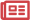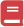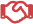# Weld Heat Formula

The general heat generation formula for resistance welding is:

Heat = Ix R x t x K

Where ‘I’ is the weld current through the workpieces, ‘R’ is the electrical resistance (in ohms) of the workpieces, ‘t’ is the weld time (in hertz, milliseconds or microseconds), and ‘K’ is a thermal constant. The weld current (I) and duration of current (t) are controlled by the resistance welding power supply. The resistance of the workpieces (R) is a function of the weld force and the materials used. The thermal constant ‘K’ can be affected by part geometry, fixturing and weld force.#### News and Events

Visit the Newsroom#### Learning Center